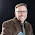Thursday, November 17, 2011

Elementary Algebra (Ch.4) Solving Linear Systems

Elementary Algebra is an open textbook that is available to read for free on the flatworld knowledge website. Chapter 4 is an introduction to solving linear systems of equations and inequalities. In this chapter, we learn what it means to solve of equations geometrically, including dependent systems. In addition, we thoroughly cover the substitution and elimination methods.

4.1 Solving Linear Systems by Graphing
4.2 Solving Linear Systems by Substitution
4.3 Solving Linear Systems by Elimination
4.4 Applications of Linear Systems
4.5 Solving Systems of Linear Inequalities

You will find a complete review exercise section and a practice test.

4.6 Review Exercises and Sample Exam

Feel free to copy and paste the links found here and post them in your LMS. Links to all of the chapters can be found by clicking the Elementary Algebra course button at the top of this page.

---1.Solving linear equations?
It says I have to solve

-3k + 4 = 8k - 15 - 6k - 11

On the problem, do I combine like terms on one side first? Like the 8k and the 6k? If so do I add them or subtract them?

Can someone please show me the exact steps for doing this? Thanks

2.I like to add or subtract "same side" like terms first.

-3k + 4 = 2k - 26

Then add or subtract "opposite side" like terms. Here subtract 4 on both sides and subtract 2k on both sides.

-5k = -30

Lastly, divide by sides by -5.

k = 6

Click Show steps in Wolfram Alpha: http://www.wolframalpha.com/input/?i=solve+-3k+%2B+4+%3D+8k+-+15+-+6k+-+11